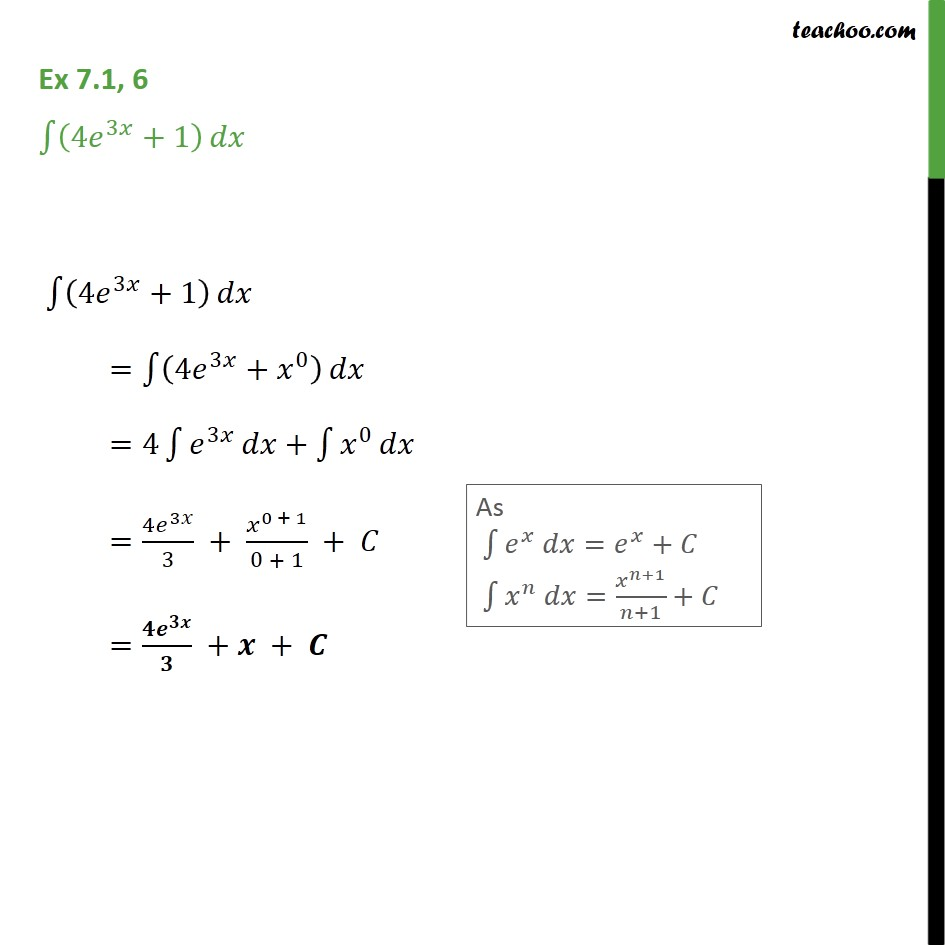Ex 7.1

Chapter 7 Class 12 Integrals
Serial order wiseLearn in your speed, with individual attention - Teachoo Maths 1-on-1 Class

### Transcript

Ex 7.1, 6 - Chapter 7 Class 12 Integrals - NCERT Book ∫ (4e^3x + 1) dx ∫ (4e^3x + 1) dx =∫ (4 e^3x + x^0) dx =4∫1▒𝑒^3𝑥 𝑑𝑥+∫1▒𝑥^0 𝑑𝑥 =(4𝑒^3𝑥)/3 + 𝑥^(0 + 1)/(0 + 1) + 𝐶As ∫1▒〖𝑒^𝑥 𝑑𝑥=𝑒^𝑥+𝐶〗 ∫1▒〖𝑥^𝑛 𝑑𝑥=𝑥^(𝑛+1)/(𝑛+1)+𝐶〗 =(𝟒𝒆^𝟑𝒙)/𝟑 +𝒙 + 𝑪